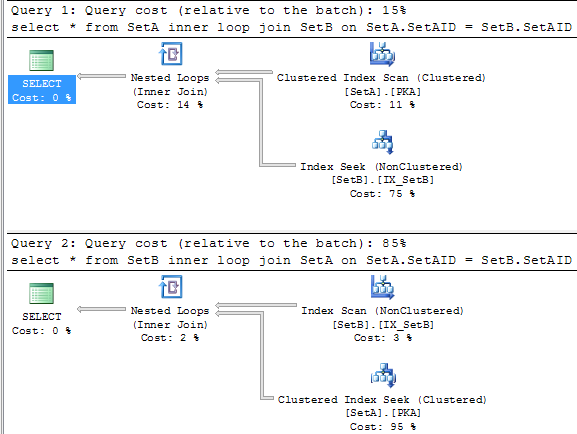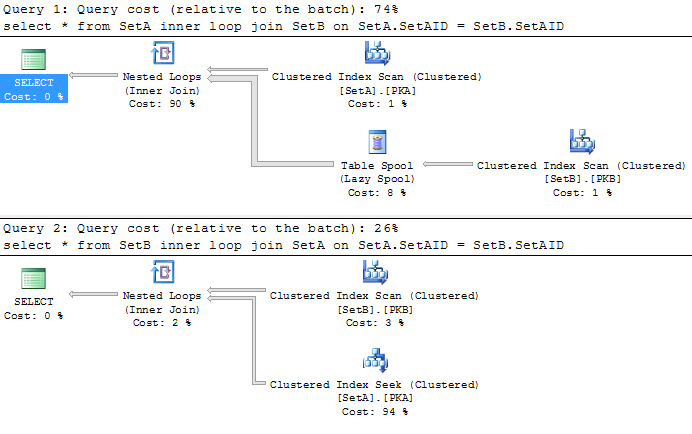As we know that Nested Loop is a physical operator which can perform different kind of logical joins between 2 sets, SetA and SetB for instance. Does SetA joining SetB equal to SetB joining SetA from perfromance perspective(assume 2 sets have enough indexes)? It depends?

I would say NO in general. People who say “depends” are talking about few special cases. From Nested Loop itself, there are no differences on what are in the outer input and what are in the inner input. It always estimate 4.2 (4.18) microseconds to perform a comparison between those 2 inputs for records from each. However, Nested Loop has to have 2 inputs which are coming from sets. Cost of accessing records from those 2 sets are different.

Let’s say SetA(SetAID int) has 100 records and SetB(SetBID, SetAID) has 1000 records. They are joining by Joining Keys SetA.SetAID and SetB.SetAID. Each records in SetA has 10 child records in SetB.

```use tempdb
set statistics xml off
go
if object_id('SetA') is not null
drop table SetA;

create table SetA(SetAID int, constraint PKA primary key(SetAID))
go
if object_id('SetB') is not null
drop table SetB;
create table SetB(SetBID int, SetAID int, constraint PKB primary key(SetBID))
create index IX_SetB on SetB(SetAID)
go
set nocount on
declare @i int, @j int
select @i = 1
while @i <= 100
begin
insert into SetA values(@i)
select @j = 0
while @j<= 9
begin
insert into SetB values(@i*10 + @j, @i)
select @j = @j + 1
end
select @i = @i + 1
end
go
update statistics SetA
update statistics SetB
set statistics xml on
go
select *
from SetA
inner loop join SetB on SetA.SetAID = SetB.SetAID
select *
from SetB
inner loop join SetA on SetA.SetAID = SetB.SetAID
set statistics xml off```The first query only takes 15% of total cost where the second one takes 85% of total. The second query is more than 5 times slower than the first one! Why is that? let’s do a calculation manually.

The First Query

Accessing SetA is using clustered primary key scan, the costs are
CPU Cost = 0.1581 ms + 0.0011 * 99 = 0.267 ms
IO Cost = 3.125 ms + 0.7407 * 0 = 3.125ms ( the table is small enough which can fit in one data page)
Total Cost of the Operator = 0.267 + 3.125 = 3.392

Cost of Nested Loop = 0.00418ms * 1000 = 4.18 ms

SetB will be accessed 100 times. Each time it will return 10 records logically. It uses none clustered index on SetAID column. The cost of it will be

Initial cost:
CPU Cost = 0.1581 ms + 0.0011 * 9 = 0.168 ms
IO Cost = 3.125 ms + 0.7407 * 0 = 3.125ms
Total Initial Cost = 0.168 + 3.125 = 3.293 ms

If you look at the property of this operator, you will see Estimated Rebinds is 99. This means that the same searching algorithm will have new parameter rebinded 99 times. As we know that SetB will use 2 pages. In this case those 2 pages will all be accessed. So additional cost on IO will be counted. The calculation here is little bit differently:

Cost for accessing the second page will be IO Cost, IO Cost = 3.125 ms
Cost for rebinding will be CPU cost, CPU Cost = (0.1581 ms + 0.0011 * 9) * 99 = 16.632
Total Cost of the Operator = 3.293 + 3.125 + 16.632 = 23.05 ms

Cost of the First query = 3.392 + 4.18 + 23.05 = 30.622 ms.

The Second Query
The size of the record in SetB is over 8 bytes. After adding up the overhead in the storage engine, such as record tag, it will be more than 10, in which, it will need 2 data pages to hold the 1000 records.

SQL Server accesses SetB using none clustered index scan rather than clustered index scan. The cost of this operator will be simple

CPU Cost = 0.1581 ms + 0.0011 * 999 = 1.257 ms
IO Cost = 3.125 ms + 0.7407 * 1 = 3.8657 ms
Total Cost of the operator = 1.257 + 3.8657 = 5.1227 ms

Cost of Nested Loop = 0.00418ms * 1000 = 4.18 ms

Table SetA in this case will be accessed 1000 times. Each time it will return 1 records. The initial cost for accessing SetA will be

Initial Cost:
CPU Cost = 0.1581 ms + 0.0011 * 0 = 0.1581 ms
IO Cost = 3.125 ms + 0.7407 * 0 = 3.125 ms
Total Initial Cost = 0.1581 + 3.125 = 3.2831 ms

According to the query plan, SQL Server does 990 rebinds with 9 rewinds on SetA
Cost of the Rebinds = 0.1581 * 990 = 156.519 ms
Cost of the Rewinds = 0.1581 * 9 = 1.4229 ms
Cost of the Operator = 3.2831 + 156.519 + 1.4229 = 161.225 ms

Total cost of the Second query = 5.1227 + 4.18 + 161.225 = 170.5277

Over all percentage of the first query = 30.622 * 100 /(30.622 + 170.5277) = 15.223%
Over all percentage of the first query = 170.5277 * 100 /(30.622 + 170.5277) = 84.777%What will happen if we remove the index IX_SetB from SetB table? The query plan will become to the picture right. The first query scans both SetA and SetB where SetB is buffered by Table Spool operator. While Nested Loops are being executed, each value from the outer input will be compared with all the records in the buffer (spool). Comparison will repeat 100 times as you can see 99 rewinds on the Spool operator. Each scan, inner join has to compare 1000 times to get the matched values. This means that The Nested Loop performs 100*1000 comparisons. Each comparision costs 0.00418 ms. Cost of all comparisons will be 0.00418 * 100 * 1000 = 418 ms (close to half second). This makes Nested Loop the most expensive operator in the first query. The second query becomes to the faster one.

Wrap-up, few things about Nested Loop,

1. The output order of outer table is not important. But number of records returned from outer table is important. Ensure you have proper filters on the outer table to eliminate number of records. To optimizing an outer table, you only need to consider the filters are Search-able ( have index on the filtered column)
2. Number of record returned from inner table is less important than having index on joining keys. The index stagegy for inner table will be joining keys – filter keys
3. SQL Server is using statistics on both tables to determine which one should be the outer one. Sometime, SQL Server will place the table that you think it should be outer to inner.

P.S. none of the numbers above from official doucments. They might not be 100% accurate all the time.

Query Plan (08) – Nested Loop Cont.

#### You May Also Like

This site uses Akismet to reduce spam. Learn how your comment data is processed.